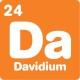# What's new## Square and cube number brainteaser

, 2 May 2018

When you multiply a whole number by itself, you get a square number. When you take three copies of a whole number and multiply them all together, you get a cube number.

There’s only one two digit number that is both a square and a cube. Can you work out what number it is?

For those looking for a challenge, can you find the only three digit number that is both a square and a cube?There are plenty of ways to do this puzzle. One way is to list out all the square numbers with two digits: 16, 25, 36, 49, 64, 81.

Then list out all the cube numbers with two digits: 27, 64.

The answer is the only number in both lists: 64.

There’s a trickier way to solve this puzzle too, which makes it easier to solve the challenge question. It relies on a maths fact: if you multiply two squares together, you get a square! If you’re not sure about this fact, try a few examples to see if you can work out what’s going on.

We’re going to start with a square, and use it to make a square that is also a cube. Let’s start with a small square: 4.

We know 4 x 4 = 16 is a square from the fact above, and also because of what a square means. But we can go one step further. 4 x 16 = 64 is the product of two squares, so it is also a square. But you can also write it as 64 = 4 x 4 x 4, making it a cube.

Using this method we can work out the next square that is a cube by repeating this process. This time, start with the next smallest square: 9. First, 9 x 9 = 81.

9 x 81 = 729, is the product of two squares, so it is also a square. You can also write it as 729 = 9 x 9 x 9, making it a cube.

Therefore the answer to the challenge is 729.

For more brainteasers and puzzles for kids, subscribe to Double Helix magazine!Categories:

## Similar posts

1.The first square and cube number when doubled is

2.It is useful for me but I can not understand the second one (729)

1.hi there!
It’s a bit tricky, but the idea is:
3 x 3 x 3 x 3 x 3 x 3 = 729
and we can group those to make three terms:
3×3   x   3×3   x   3×3 = 729
so it’s a cube

We can also group them to make two terms:
3x3x3   x   3x3x3 = 729
so it’s a square too!

3.So you don’t understand
Let me explain in short
See 4³=64, 8²=64
Now:- 9³=729, 27²=729
This also works with all the squares like 16 cube and 81 cube

4.I found a little formula for it
So if x³=y then do
((√x)³)² =y and here it is the square of it

5.But sqrt(x) cubed squared = x cubed because sqrt and squared cancel each other so it’s the same this as (x to the power of 3) = y

6.is it right 16^3=64^2

1.That’s definitely a square and cube number – and it’s the only one with 4 digits!